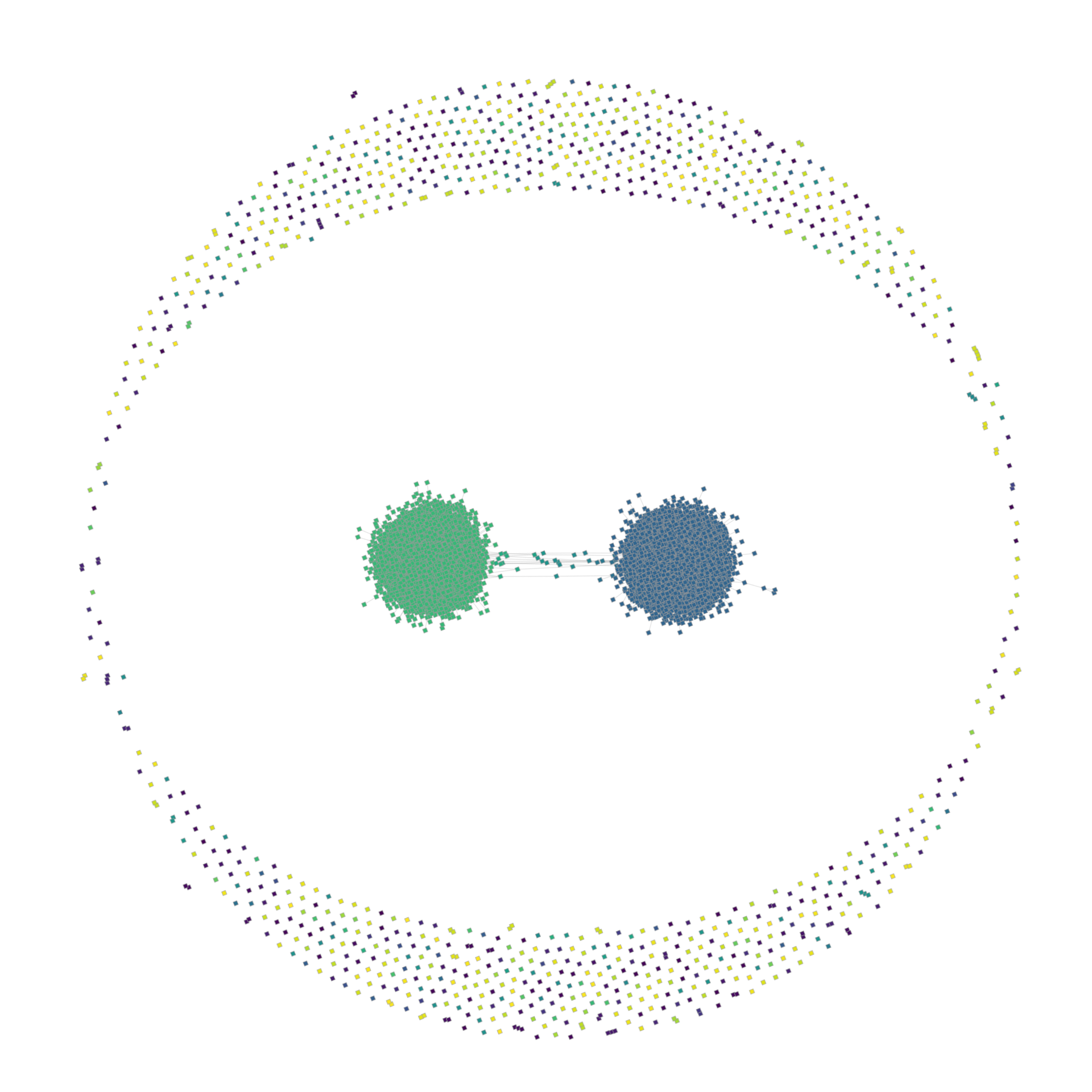Use the right tool for the job. In my everyday life, this means writing simulations in Rust and visualize the results in Python. Thanks to inline-python this process works extremely well.

use inline_python::python;

fn main() {
let x: Vec<f32> = (0..628).map(|i| i as f32 / 100.).collect();
let y: Vec<f32> = x.iter().map(|x| x.sin()).collect();

python! {
import numpy as np
from matplotlib import pyplot as plt

plt.plot('x, 'y)
plt.show()
}
}


This minimal example is useless of course. But I already used this crate productively to simulate dynamics on a petgraph graph and visualize its state via graph-tool.